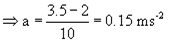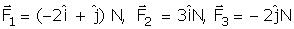Courses

# Test: Newton’s Second Law of Motion

## 10 Questions MCQ Test Physics Class 11 | Test: Newton’s Second Law of Motion

Description
This mock test of Test: Newton’s Second Law of Motion for JEE helps you for every JEE entrance exam. This contains 10 Multiple Choice Questions for JEE Test: Newton’s Second Law of Motion (mcq) to study with solutions a complete question bank. The solved questions answers in this Test: Newton’s Second Law of Motion quiz give you a good mix of easy questions and tough questions. JEE students definitely take this Test: Newton’s Second Law of Motion exercise for a better result in the exam. You can find other Test: Newton’s Second Law of Motion extra questions, long questions & short questions for JEE on EduRev as well by searching above.
QUESTION: 1

### A constant force acting on a body of mass 3 kg changes its speed from 2 m/s to 3.5 m/s in 10 second. If the direction of motion of the body remains unchanged, what is the magnitude and direction of the force?

Solution:

Given : Mass, m = 3 kg;

Initial Velocity, u = 2m/s;

Final velocity, v = 3.5 m/s

Time taken, t = 10 seconds

v = u+at

3.5 = 2 +a x 10F = ma = 3 x 0.15 = 0.45 N

Since the applied force increase the speed of the body, it acts along the direction of motion.

QUESTION: 2

### The product of mass and velocity is known as

Solution:

The product of velocity and mass is known as momentum which is a vector quantity.

QUESTION: 3

### In cgs system the gravitational unit of force is gram weight (g wt).Then 1g wt (or g f) =_______ dyne.

Solution:

For SI to CGS conversion,

1 Kg = 103 g

1 m = 102 cm

=> In CGS System,

1 N (Kg.m/s2) = 105 dynes (g.cm/s2)

=> 1 g.wt = 9.8 m/s2 x 102 cm / 1 s2 x 10-3 g x 10-2 cm / 1 s x 105 dynes

= 980 dynes (g.cm/s2)

QUESTION: 4

The dimensional formula of Plancks’s constant and angular momentum are

Solution:

Planck's Constant (h)  = 6.626176 x 10-34  m2 kg/s
So, Unit of planck constant=  m2 kg/s

Dimensions =M L2 T −1   ________ (1)

Angular momentum l = mvr

Where, m-mass

v-velocity

Dimensions of angular momentum  =  M L T −1 L   = M  L2  T −1  _______________ (2)
From (1) and (2).

Planck's constant and angular momentum have the same dimensions.

QUESTION: 5

Which of the following quantity has the unit of newton-second?

Solution:

Net external force F = dp/dt, so using dimensional analysis, unit of momentum should be the same as unit of force times unit of time which is Newton second.

QUESTION: 6

If newton is the unit of force in MKS system then, in CGS system 1N= ______ dyne

Solution:

1N = kg m /sec
1 dyne = gram cm/sec
Thus 1N in CGS system = 1000 gram 100 cm/sec
That is 1N = 100000 dyne

QUESTION: 7

Three forcesact on an object of mass m = 2 kg. The acceleration of the object in m/s2 is:

Solution:

Force vector follows the principle of superposition which says all the force vectors can be vectorially added if applied on one point to get the net force vector. Hence we get
F = F1 + F2 + F3
= (-2 + 3) i + (1 - 2) j
F = i - j = ma
Thus we get a = (i - j) /m
= (i - j) / 2

QUESTION: 8

Newton’s second law of motion relates

Solution:

Newton's 2nd Law of motion states that rate of change of momentum is directly proportional to external force applied. Hence, it relates rate of change of momentum to external force applied.

QUESTION: 9

A cyclist of mass 30 kg exerts a force of 250 N to move his cycle. The acceleration is 4 ms−2. The force of friction between the road and tyres will be

Solution:

F = ma so a= 250/30

a = 8.34

now the result is 4mt/secsqure so reduction is 4.34

so friction force will b 4.34 ×30

F is 130.2 n

QUESTION: 10

The time rate of change of momentum is called:

Solution:

Time rate of change of angular momentum represents torque. Hence force is known as rate change of momentum. The rate of change of momentum is called force by Newton's second law of motion.